# The 48 Special Crystal Forms

Steven Dutch, Professor Emeritus, Natural and Applied Sciences, University of Wisconsin - Green Bay

## Forms, Open and Closed

Any group of crystal faces related by the same symmetry is called a form. There are 47 or 48 crystal forms depending on the classification used.

Closed forms are those groups of faces all related by symmetry that completely enclose a volume of space. It is possible for a crystal to have faces entirely of one closed form. Open  forms are those groups of faces all related by symmetry that do not completely enclose a volume of space. A crystal with open form faces requires additional faces as well. There are 17 or 18 open forms and 30 closed forms.

## Triclinic, Monoclinic and Orthorhombic Forms

Pedion
A single face unrelated to any other by symmetry. Open
Pinacoid
A pair of parallel faces related by mirror plane or twofold symmetry axis. Open
Dihedron
A pair of intersecting faces related by mirror plane or twofold symmetry axis. Some crystallographers distinguish between domes (pairs of intersecting faces related by mirror plane) and sphenoids (pairs of intersecting faces related by twofold symmetry axis). All are open forms
Pyramid
A set of faces related by symmetry and meeting at a common point. Open form.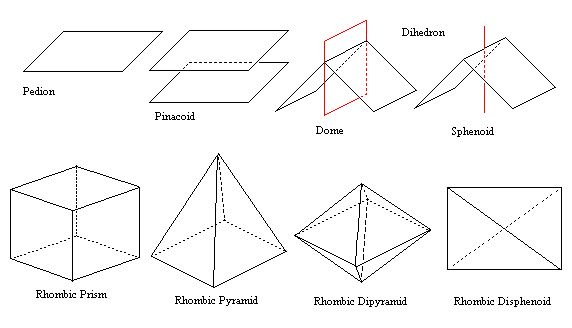## 3-, 4- and 6-Fold Prisms

Prism
A collection of faces all parallel to a symmetry axis. All are open.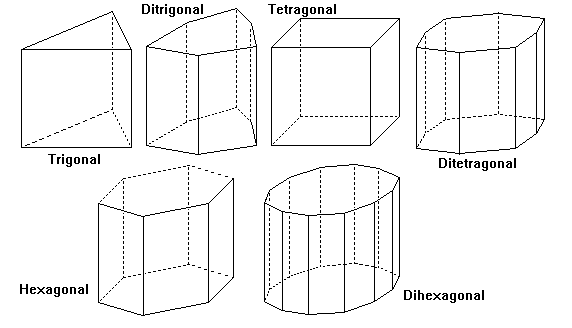## 3-, 4- and 6-Fold Pyramids

Pyramid
A group of faces intersecting at a symmetry axis. All are open. The base of the pyramid would be a pedion.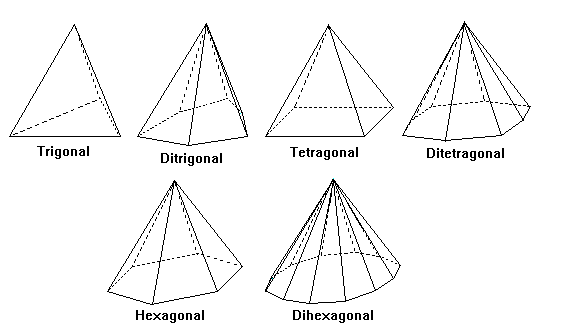## 3-, 4- and 6-Fold Dipyramids

Dipyramid
Two pyramids joined base to base along a mirror plane. All are closed, as are all following forms.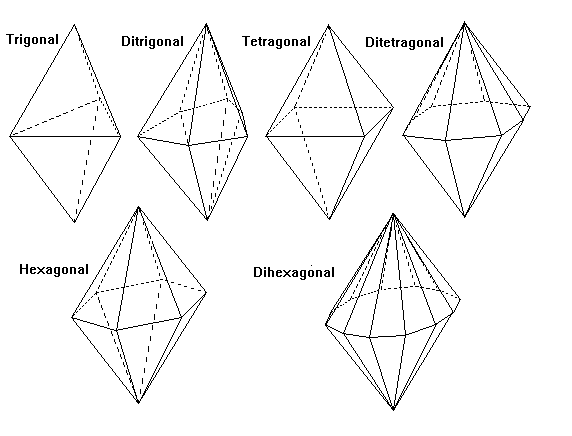## Scalenohedra and Trapezohedra

Disphenoid
A solid with four congruent triangle faces, like a distorted tetrahedron. Midpoints of edges are twofold symmetry axes. In the tetragonal disphenoid the faces are isoceles triangles and a fourfold inversion axis joins the midpoints of the bases of the isoceles triangles.
Scalenohedron
A solid made up of scalene triangle faces (all sides unequal)
Trapezohedron
A solid made of trapezia (irregular quadrilaterals)
Rhombohedron
A solid with six congruent parallelogram faces. Can be considered a cube distorted along one of its diagonal three-fold symmetry axes.## Tetartoidal, Gyroidal and Diploidal Forms

Tetartoid
The general form for symmetry class 233. 12 congruent irregular pentagonal faces. The name comes from a Greek root for one-fourth because only a quarter of the 48 faces for full isometric symmetry are present.
Gyroid
The general form for symmetry class 432. 24 congruent irregular pentagonal faces.
Diploid
The general form for symmetry class 2/m3*. 24 congruent irregular quadrilateral faces. The name comes from a Latin root for half, because half of the 48 faces for full isometric symmetry are present.
Pyritohedron
Special form (hk0) of symmetry class 2/m3*. Faces are each perpendicular to a mirror plane, reducing the number of faces to 12 pentagonal faces. Although this superficially looks like the Platonic solid with 12 regular pentagon faces, these faces are not regular.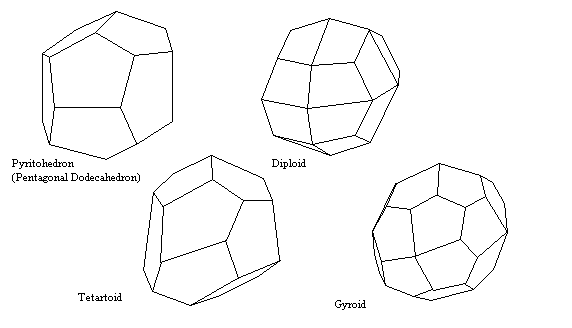## Hextetrahedral Forms

Tetrahedron
Four equilateral triangle faces (111)
Trapezohedral Tristetrahedron
12 kite-shaped faces (hll)
Trigonal Tristetrahedron
12 isoceles triangle faces (hhl). Like an tetrahedron with a low triangular pyramid built on each face.
Hextetrahedron
24 triangular faces (hkl) The general form.## Hexoctahedral Forms

Cube
Six square faces (100).
Octahedron
Eight equilateral triangle faces (111)
Rhombic Dodecahedron
12 rhombic faces (110)
Trapezohedral Trisoctahedron
24 kite-shaped faces (hhl). Note that the Miller indices for the two trisoctahedra are the opposite of those for the tristetrahedra.
Trigonal Trisoctahedron
24 isoceles triangle faces (hll). Like an octahedron with a low triangular pyramid built on each face.
Tetrahexahedron
24 isoceles triangle faces (h0l). Like an cube with a low pyramid built on each face.
Hexoctahedron
48 triangular faces (hkl) The general form